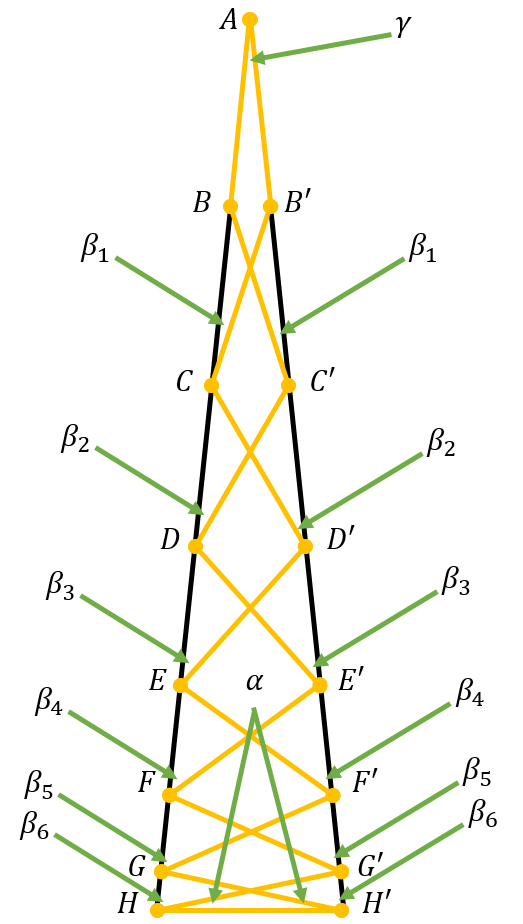# Triangle with $q$ identical linesThe task is: Given a triangle with $q$ identical lines, in this way as you can see on the picture. The orange lines are the same. Calculate $\gamma$!

$\Delta ABC'$ is an isosceles triangle, therefore $\beta_1=\gamma$.

The sum of the angles at the B point is $180^{\circ}$, because AH is a line: $\cancel{180^{\circ}}-2\gamma+\beta_2=\cancel{180^{\circ}}$ $\beta_2=2\gamma$

From the isosceles triangles and the sum of angles at the C point: $\beta_1+\cancel{180^{\circ}}-2\beta_2+\beta_3=\cancel{180^{\circ}}\implies \beta_3=4\gamma-\gamma =3\gamma$ Same way $\beta_4=4\gamma, \beta_5=5\gamma, \cdots, \beta_n=n\gamma$, because if we see an angle: $\beta_{n-2}+\cancel{180^{\circ}}-2\beta_{n-1}+\beta_n=\cancel{180^{\circ}}\implies \beta_n=2\beta_{n-1}-\beta_{n-2}=(2(n-1)-n+2)\gamma=n\gamma$ This is true for the first, second and third angles, so this must be true for every angles.

If we have a triangle with $q$ lines, then the last $\beta$ is the $\frac{q-3}{2}$th.

Now in the bottom $HG'H'$ triangle: $\alpha+\alpha+\alpha+\beta_6+\beta_6=180^{\circ}$ or generally $3\alpha+2\beta_{\frac{q-3}{2}}=180^{\circ}$. But if we see the big triangle $2\alpha+2\beta_{\frac{q-3}{2}}+\gamma=180^{\circ}$. Therefore $\alpha=\gamma$. After substituting: $3\gamma+2\beta_{\frac{q-3}{2}}=180^{\circ}$. Replacing $\beta_{\frac{q-3}{2}}$: $3\gamma+2\cfrac{q-3}{2}\gamma=180^{\circ}$ $3\gamma+q\gamma-3\gamma=180^{\circ}$ $q\gamma=180^{\circ}$ $\boxed{\gamma=\cfrac{180^{\circ}}{q}}$

Now can you solve this?Note by A Former Brilliant Member
1 year ago

This discussion board is a place to discuss our Daily Challenges and the math and science related to those challenges. Explanations are more than just a solution — they should explain the steps and thinking strategies that you used to obtain the solution. Comments should further the discussion of math and science.

When posting on Brilliant:

• Use the emojis to react to an explanation, whether you're congratulating a job well done , or just really confused .
• Ask specific questions about the challenge or the steps in somebody's explanation. Well-posed questions can add a lot to the discussion, but posting "I don't understand!" doesn't help anyone.
• Try to contribute something new to the discussion, whether it is an extension, generalization or other idea related to the challenge.

MarkdownAppears as
*italics* or _italics_ italics
**bold** or __bold__ bold
- bulleted- list
• bulleted
• list
1. numbered2. list
1. numbered
2. list
Note: you must add a full line of space before and after lists for them to show up correctly
paragraph 1paragraph 2

paragraph 1

paragraph 2

[example link](https://brilliant.org)example link
> This is a quote
This is a quote
    # I indented these lines
# 4 spaces, and now they show
# up as a code block.

print "hello world"
# I indented these lines
# 4 spaces, and now they show
# up as a code block.

print "hello world"
MathAppears as
Remember to wrap math in $$ ... $$ or $ ... $ to ensure proper formatting.
2 \times 3 $2 \times 3$
2^{34} $2^{34}$
a_{i-1} $a_{i-1}$
\frac{2}{3} $\frac{2}{3}$
\sqrt{2} $\sqrt{2}$
\sum_{i=1}^3 $\sum_{i=1}^3$
\sin \theta $\sin \theta$
\boxed{123} $\boxed{123}$

Sort by:

Nice proof! Explains where I went wrong! @Páll Márton.

- 1 year ago

Thank you! Did you solve my problem?

No! That's why I said it explains where I went wrong.

- 1 year ago

Angles, not angels.

- 1 year ago

Yeah. I use Word2016 to write the text and I just paste them there, but the default language isn't English so the autocorrector "corrects" my "typos".

I will add this word to the Word. Last time it wrote mooves instead of moves

Oh. I see. Doesn't happen with me though.

Also, tip:

If you wish to delete a problem, copy and paste the solution in Word, then copy the problem, go to create a new problem (open a new tab for this), paste the original problem's text, copy the title, paste it in the title section, change the answers (if needed) - if not, press Post. Then, copy the solution from Word, paste it and make any corrections (if needed) - if not, press Preview and Submit. Then close the Word Document.

- 1 year ago

Or I will use txt

Up to you.

I used this technique for my Hexadecimal Equations Problem $6$ up to Version $4$.

- 1 year ago

this note isn't very famous I think you can continue this :)

I will continue the prove chain. (albeit later)

Also, what if (for your next problem involving this):

$\frac{180}{3600}$$= 0.05$ degrees

- 1 year ago

I don't understand

Triangle with $3600$ identical lines?

- 1 year ago

Ohh. q is odd :)

Else we can get only an intervallllll

Triangle with $3601$ identical lines?

$\frac{180}{3601}$$= 0.049986115$ degrees

Also, how do you do the degree sign? Baffles me...

P.S. Check out my note - Interesting Thing about Multiplication Last Digit Sequences (Even)

- 1 year ago

Yeah. You just shifted the 2, 4, 6 and 8. ^{\circ}

What about the triangle with $3601$ identical lines?

- 1 year ago

I think with 45 lines was enaugh. If somebody can solve with 45, then he/she can solve with 3601 too.

You mean enough, right?

- 1 year ago

Yeah. This was my mistake :) English is too hard. To write from pronunciation is hard

Excellent exposition.

- 1 year ago

Thank you!

Wow! Good proof!

- 1 year ago

Thank you!

Excellent Proof @Páll Márton.

- 1 year ago